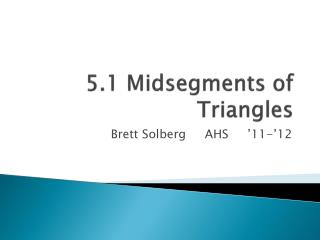Download Presentation5.1 Midsegments of Triangles5.1 Midsegments of Triangles - PowerPoint PPT Presentation

Download Presentation5.1 Midsegments of Triangles
An Image/Link below is provided (as is) to download presentation

Download Policy: Content on the Website is provided to you AS IS for your information and personal use and may not be sold / licensed / shared on other websites without getting consent from its author. While downloading, if for some reason you are not able to download a presentation, the publisher may have deleted the file from their server.

- - - - - - - - - - - - - - - - - - - - - - - - - - - E N D - - - - - - - - - - - - - - - - - - - - - - - - - - -
Presentation Transcript

1. 5.1 Midsegments of Triangles Brett Solberg AHS ’11-’12

2. Warm-up 11-28-2011 • 1) Find the distance between (1, 4) and (4,8). • 2) Find the midpoint of the segment whose endpoints are (4, 11) and (6, 3). • 3) Find the slope of the line containing the points (8, 3) and (7, 12).

3. Today’s Agenda • Chapter 5 Relationships Within Triangles • Triangle Midsegments • Test Make-up

4. Midsegment of a Triangle • A line that connects two midpoints of two sides of a triangle is called the midsegment of a triangle. • DE is a midsegment of ∆ABC

5. Discovery • What relationships did you discover between a triangle midsegment and the third side of a triangle?

6. Triangle Midsegment Theorem • The midsegment of a triangle is half the length of the third side of a triangle. • DE = 5 • BC = 10

7. Triangle Midsegment Theorem • The midsegment of a triangle is parallel to the third side of a triangle. • DE || BC

8. Example 1 • Which segments are parallel?

9. Example 3 • M, N, and P are midpoint in ∆XYZ. The perimeter of ∆MNP is 60. Find NP and YZ. • MN = 22 MP = 24 • MN + MP + NP = 60 • 22 + 24 + NP = 60 • NP = 14 • XY = 28 • YZ = 44 • XZ = 48

10. Homework • 5.1 Worksheet • pg 262 #1 – 13 all

11. #1 • CD = 4 cm MO = • CE = 8 cm NO = • DE = 7 cm NM =

12. #7 • Find the value of the variable. • Perimeter of ∆ABC = 32 cm • n + 7/8n + 1/2n = 32 • 8n + 7n + 4n = 256 • 19n = 256 • n = 13.47